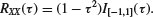PROBLEMS

Ergodicity

8.1 (a) Derive the necessary and sufficient condition in (8.23) for random sequence X[k] to be ergodic in the mean. (b) Verify the sufficient condition in (8.24).

8.2 Let X[k] have zero mean and autocovariance function CXX[m] = α |m|. Determine if both conditions in the first problem are satisfied for this random sequence.

8.3 Suppose that X(t) is ergodic in the mean, and let Y be a random variable that is independent of X(t). Define random process Z(t) = YX(t) and determine if it is also ergodic in the mean. Note that a realization is of the form z(t) = yx(t) such that outcome y remains fixed with time as x(t) varies.

8.4 Random process X(tx) has zero mean and the following autocorrelation function:

(8.332)Determine if X(t) is ergodic in the mean.

8.5 Random process X(t) has the following periodic autocovariance function:

(8.333)for some fixed frequency fc. Determine if X(t) is ergodic in the mean.

8.6 For RXX(τ) = exp(−|τ|), use the result in Example 8.3 to determine if the zero-mean Gaussian random process X(t) is ergodic in correlation.

Power Spectral Density

8.7 For the cross-PSD, show that (a) SXY(f) = SYX(−f) and (b) the imaginary part is an odd function.

8.8 Consider the following random process:

(8.334)

where Φ is uniformly distributed on . Find ...

Get Probability, Random Variables, and Random Processes: Theory and Signal Processing Applications now with the O’Reilly learning platform.

O’Reilly members experience books, live events, courses curated by job role, and more from O’Reilly and nearly 200 top publishers.